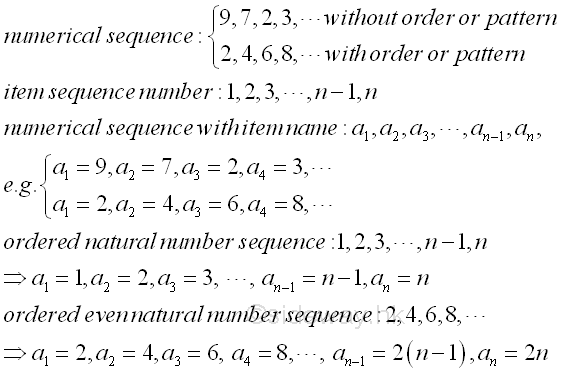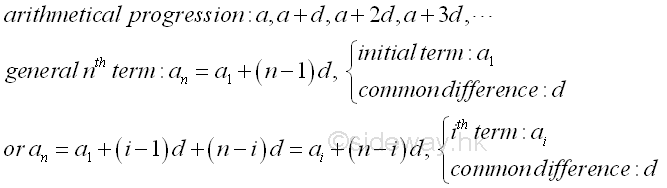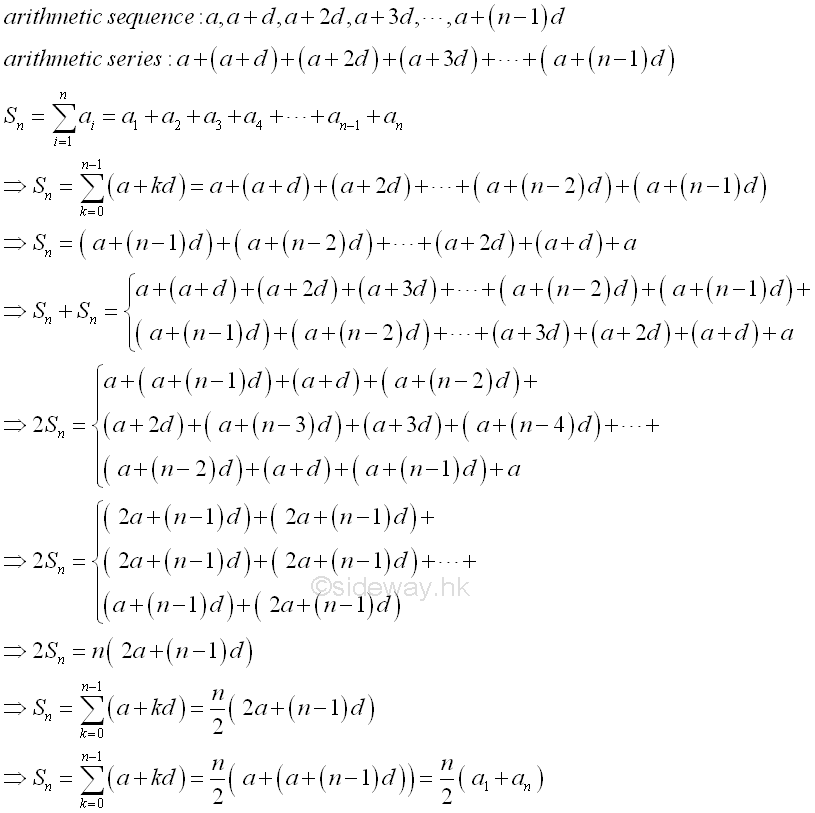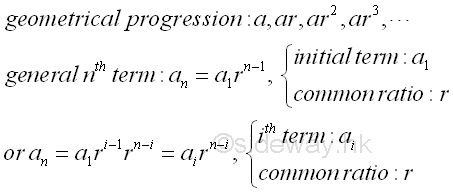output.to from Sideway
Draft for Information Only

# Content

```  Numerical Sequences  Arithmetical Progression (A.P.)  Arithmetic Series  Geometrical Progression (G.P.)  Geometric Series```

## Numerical Sequences

A numerical sequence is a list of numbers arranged in an order. The numerical sequence itself can be either arranged in a particular order or pattern, or arranged without any order or pattern. The elements of a sequence with n element  can be arranged in an ordered sequence of natural numbers with the first element equal to 1, i.e. 1, 2, 3, ..., n-1, n. The elements of a numerical sequence can therefore be represented by an item name with the corresponding item sequence number, i.e. a1, a2, a3, ..., an-1, an. If a numerical sequence is ordered with a pattern, then the elements in the numerical sequence can be expressed in term of the sequence number, n and the term un is usually called a general term of the numerical sequence. For example, the sequence of even natural numbers with sequence number n equal to the number of the element, i.e. a1=2, a2=4, a3=6, ..., an-1=2(n-1), an=2n.## Arithmetical Progression (A.P.)

Arithmetical Progression is a numerical sequence of numbers with the difference between two consecutive numbers is equal to a constant number d called common difference. The numerial sequence is of the form a, a+d, a+2d, a+3d, ... . As the arithmetical progression is a numerical sequence with a pattern, the general term of an arithmetical progression can be expressed in term of the item number, The nth term of an arithmetical progression is an=a1+(n-1)d with the initial term of an arithmetic progression, a1 and the common difference of  an arithmetic progression, d. Or in general,  an=ai+(n-i)d where ai is the ith term of an arithmetical progression.## Arithmetic Series

The sum of an arithmetical progression is called arithmetic series. Therefore an arithmetic series is of the form S=a+(a+d)+(a+2d)+(a+3d)+.... And the general format of an arithmetic series is Sn=a1+a2+a3+a4+ ....+an-1+an =a+(a+d)+(a+2d)+(a+3d)+...+(a+(n-2)d)+(a+(n-1)d) =∑ n
i=1
(ai) =∑ n-1
k=0
(a+kd). The sum of an arithmetic series can be determined by the adding the reverse order of the arithmetic series to the original arithmetic series, so that the sum of two arithmetic series can be expressed as the sum of  n equal terms of first term plus last term. And the sum of arithmetic series is therefore equal to n(2a+(n-1)d)/2. Imply## Geometrical Progression (G.P.)

Geometrical Progression is a numerical sequence of numbers with the difference between two consecutive numbers is equal to a constant multiplier number r called common ratio. The numerial sequence is of the form a, ar, ar2, ar3, ... . As the geometrcal progression is a numerical sequence with a pattern, the general term of a geometrical progression can be expressed in term of the item number, The nth term of a geometrical progression is an=a1rn-1 with the initial term of an geometric progression, a1 and the common ratio of  a geometric progression, r. Or in general,  an=airn-i where ai is the ith term of a geometrical progression.## Geometric Series

The sum of an geometrical progression is called geometric series. Therefore an geometric series is of the form S=a+ar+ar2+ar3+... . And the general format of a geometric series is Snvi8">n=a1+a2+a3+a4+ ....+an-1+an =a+ar+ar2+ar3+...+arn-2+arn-1=∑ n
i=1
(ai)=∑ n-1
k=0
(ark). The sum of a geometric series can be determined by multiplying the geometric series by the common ratio r and then deducting the result from the original geometric series, so that the difference of two difference geometric series can be expressed as the sum of the first term of the original geometric series and the last term of the new geometric series. And the sum of geometric series is therefore equal to (a(1-rn))/(1-r). ImplyIf the numerical value of the common ratio, i.e. |r| or modulus of r, is less than unity, then the sum of the geometric series to infinity can be reduced to Sn=a/(1-r). ImplyID: 130500018 Last Updated: 5/18/2013 Revision: 0 Ref:References

1. B. Joseph, 1978, University Mathematics: A Textbook for Students of Science &amp; Engineering
2. Wheatstone, C., 1854, On the Formation of Powers from Arithmetical Progressions
3. Stroud, K.A., 2001, Engineering Mathematics
4. Coolidge, J.L., 1949, The Story of The Binomial TheoremHome 5

Management

HBR 3

Information

Recreation

Culture

Chinese 1097

English 339

Reference 79Computer

Hardware 224Software

Application 213

Latex 52Manim 204

KB 1

Numeric 19

Programming

Web 289

Unicode 504

HTML 66

CSS 65

SVG 46

ASP.NET 270

OS 429

Python 72

Knowledge

Mathematics

Algebra 84

Geometry 33Calculus 67

Engineering

Mechanical

Rigid Bodies

Statics 92

Dynamics 37

Control

Natural Sciences

Electric 27